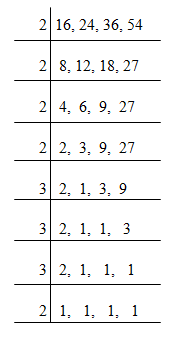# HCF and LCM

#### PREVNEXT

HCF and LCM

• Factors and Multiples: When the number ‘m’ divides another number ‘n’ exactly, then we can say that ‘m’ is a factor of ‘n’. In this case, ‘n’ is called a multiple of ‘m’.
• HCF (Highest Common Factor): The HCF of two or more than two numbers is the greatest number that divides each of them exactly. Methods to find HCF of a given number:

Factorization Method: In this each one of the given numbers is expressed as the product of prime factors. The product of least powers of common prime factors gives us HCF.

Division Method: If we want to find the H.C.F of two given numbers then divide the larger number with the smaller one. Now, divide the divisor by the remainder. Keep on repeating the process of dividing the preceding number by the remainder last obtained till zero is obtained as remainder. Then the last divisor is the required H.C.F.

L.C.M (Least Common Multiple): The least number which is exactly divisible by each one of the given numbers is called their L.C.M.

Factorization Method: In this it resolve each one of the given numbers into a product of prime factors. The product of the highest powers of all the factors gives us LCM.

Common Division Method: Arrange the numbers in a row in any order and then divide by a number which divides exactly at least two of the given numbers and carry forward the numbers which are not divisible. Just keep on repeating the above process till no two of the numbers are divisible by the same number except 1. The product of the divisors and the undivided numbers is the required L.C.M of the given numbers.

Product of two numbers = Product of their H.C.F and L.C.M

Co – primes: Two numbers are said to be co – primes if their H.C.F is 1.

H.C.F and L.C.M of Fractions:

H.C.F. = H.C.F of Numerators / L.C.M of Denominators

L.C.M. = L.C.M of Numerators / H.C.F of Denominators

H.C.F. and L.C.M. of Decimal Fractions = If the given set of numbers has decimal fractions, then first of all make the same number of decimal places by suffixing zero(s) if required. Thereafter, treat these numbers without decimal point as integers and find HCF/LCM as required. In the result, put a decimal point leaving as many digits on its right as there are in each of the numbers.

# Aptitude Questions (HCF and LCM):

1. Find the H.C.F of 108, 288 and 360.

A)    42

B)    56

C)     36

D)    60

E)     None of these

Explanation:

108 = 22 * 33

288 = 25 * 32

360 = 23 * 5 * 32

Therefore, HCF = 22 * 32 = 36.

2. Find the LCM of 16, 24, 36 and 54.

A)    543

B)    864

C)    386

D)    632

E)    None of these

Explanation:∴ LCM = 2 * 2 * 2 *2 * 3 * 3 * 3 * 2 = 864

3. The HCF of 9/10, 12/25, 18/35 and 21/40 is:

A)    3/1400

B)    3/5

C)    63/700

D)    252/5

E)    None of these

Explanation:

Required HCF = (HCF of 9, 12, 18, 21) / (LCM of 10, 25, 35, 40) = 3/1400

4. The LCM of 1/3, 5/6, 2/9 and 4/27 is:

A)    1/54

B)    10/27

C)    20/3

D)    7/45

E)    None of these

Explanation:

Required LCM = (LCM of 1, 5, 2, 4) / (HCF of 3, 6, 9, 27) = 20/3

5. The HCF of 1.25, 3.6 and 6 is:

A)    0.07

B)    0.7

C)    3.5

D)    0.25

E)    None of these

Explanation:

Given numbers with two decimal places are: 1.25, 3.60 and 6.00. Without decimal places these numbers are: 125, 360 and 600 who’s HCF is 25.

∴ HCF of given numbers = 0.25